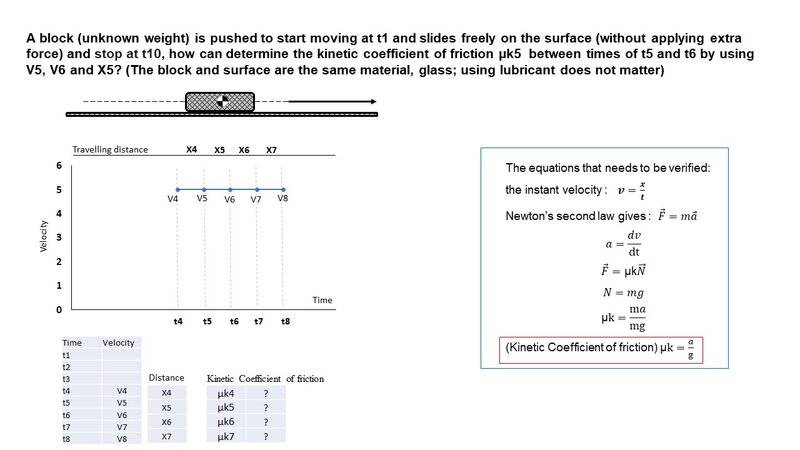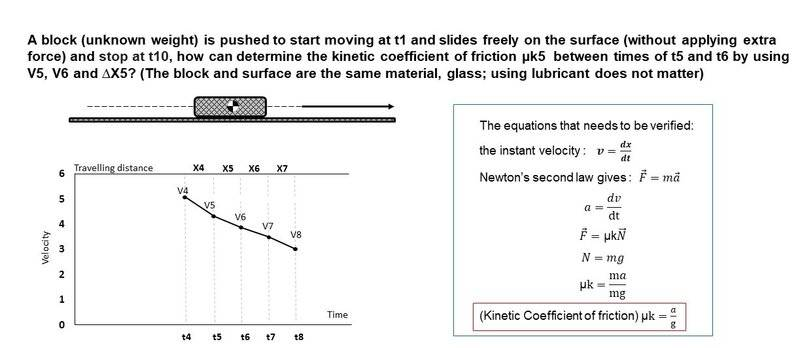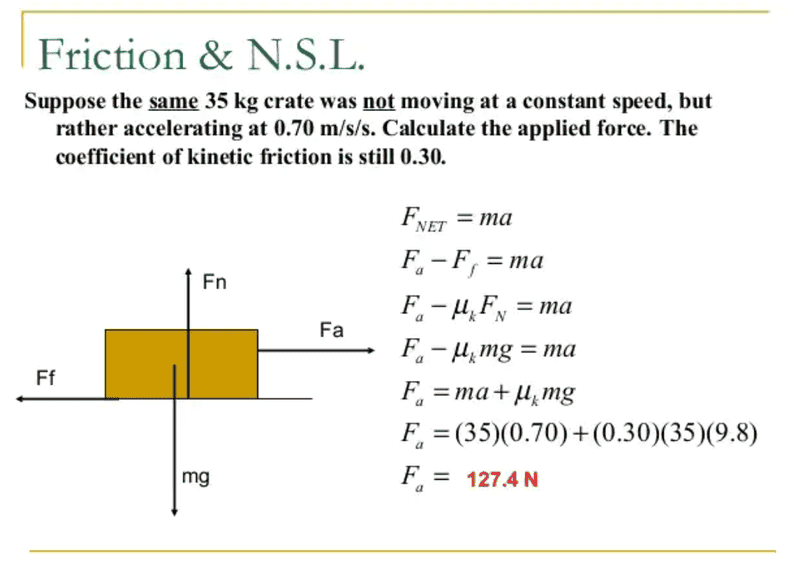# Equation calculations about the coefficient of kinetic friction

In summary, the person is asking for help calculating the coefficient of kinetic friction. They have provided equations and explained that they need help with calculating the acceleration. The person asks for help with finding a textbook or a paper that can provide a reference for the equation that they used.f

Homework Statement
A block (unknown weight) is pushed to start moving at t1 and slides freely on the surface (without applying extra force) and stop at t10, how can determine the kinetic coefficient of friction µk5 between times of t5 and t6 by using V5, V6 and X5? (The block and surface are the same material, glass; using lubricant does not matter)
Relevant Equations
µk=𝑎/g
I need an expert to verify equations about the coefficient of kinetic friction.Certainly ##\mu_k = \frac a g## is correct in this context.

•Steve4Physics
I need an expert to verify equations about the coefficient of kinetic friction.

In case you didn’t realize, I will note that @PeroK (who answered you in Post #2):
a) is an expert

1. The graph (showing v4, v5, v6, v7 and v8 on a horizontal line corresponding to v = 5) would mean the velocity is constant - which is not correct.

2. The meaning of the x-values is unclear. ambiguous as they woulld usually refer to thrFor example, is x5 the total distance traveled between times t1 and t5? Or between t4 and t5? Or between t5 and t6? Or something else?
EDIT. As noted by @jack action in Post #4, x5 is the distance covered between times t5 and t6. (Though a better symbol than 'x' might have been 'Δx'.)

3. Your equation ##v= \frac x t## is not the instantaneous velocity. The equation is generally only used to find velocity when acceleration is zero or to find average velocity.

If you can sort out the above problems, you can post your attempt at a solution and we can try to help you.

Last edited:
•PeroK
Actually, everything you've done so far is good and you are on the right path. (##\mu_k = \frac{a}{g}##)

But the problem asks to find in terms of ##v_5##, ##v_6## and ##x_5##. How can you define ##a## - between ##t_5## and ##t_6## - in those terms?

P.S.: The distance ##x_5## is clearly the traveled distance between ##t_5## and ##t_6##.

Thank you, my background is on biology, so I am sorry for poor knowledge on physics. I made the question correct, it is a simplified question from the main question.
##\mu_k = \frac{a}{g}## is the equation that can work for the main question, for verification of ##\mu_k = \frac{a}{g}## could you introduce a textbook or a paper? I need a reference for the equation that I used.Equations on the below show Fnet, Fa and Ff
I am not sure if there is missing equations that I explained above.•PeroK
Thank you, my background is on biology, so I am sorry for poor knowledge on physics. I made the question correct, it is a simplified question from the main question.
##\mu_k = \frac{a}{g}## is the equation that can work for the main question, for verification of ##\mu_k = \frac{a}{g}## could you introduce a textbook or a paper? I need a reference for the equation that I used.
View attachment 293720
Equations on the below show Fnet, Fa and Ff
I am not sure if there is missing equations that I explained above.
You know ##g## and you want to find ##\mu_k##, so you need first to calculate the acceleration ##a##. I imagine you are supposed to calculate that from the graph. You can find acceleration using either speed and position or speed and time - using the SUVAT formulas and assuming approximately constant acceleration.

Note that in this case we are talking about deceleration, so technically ##a## is the magnitude of the deceleration.

•